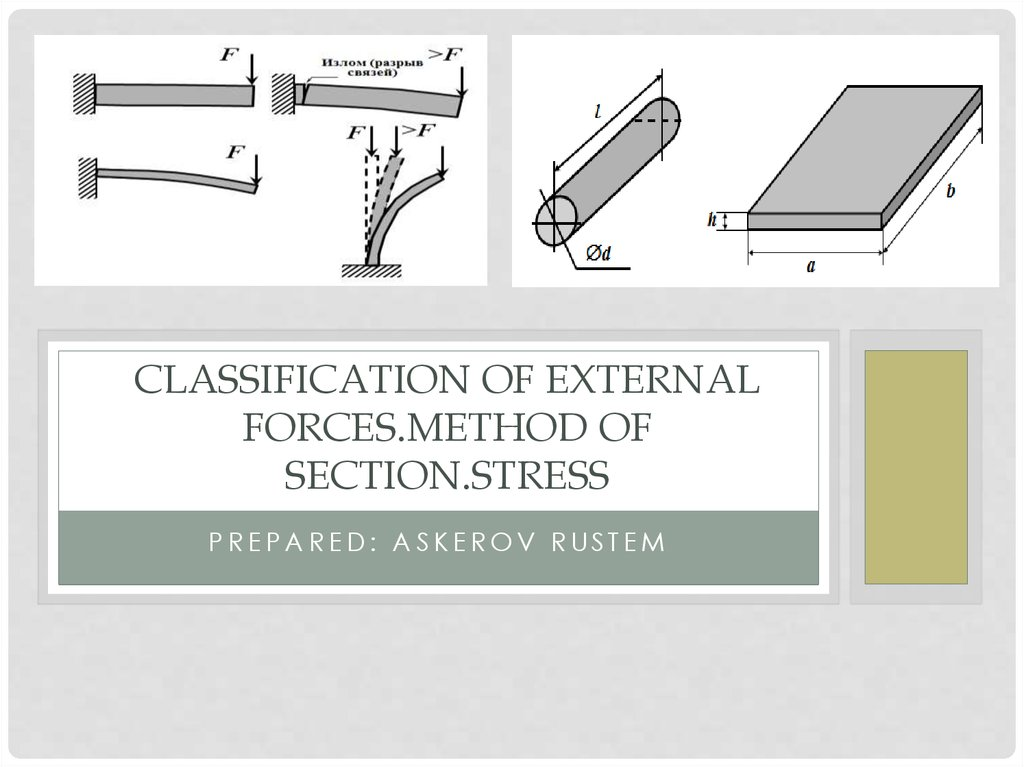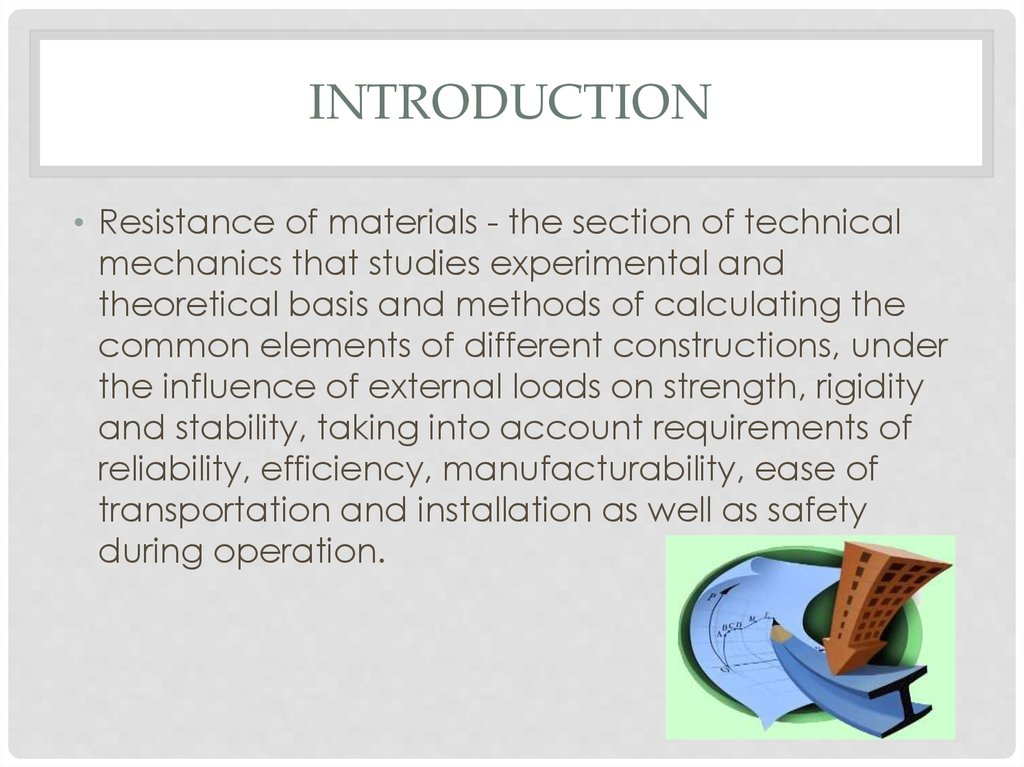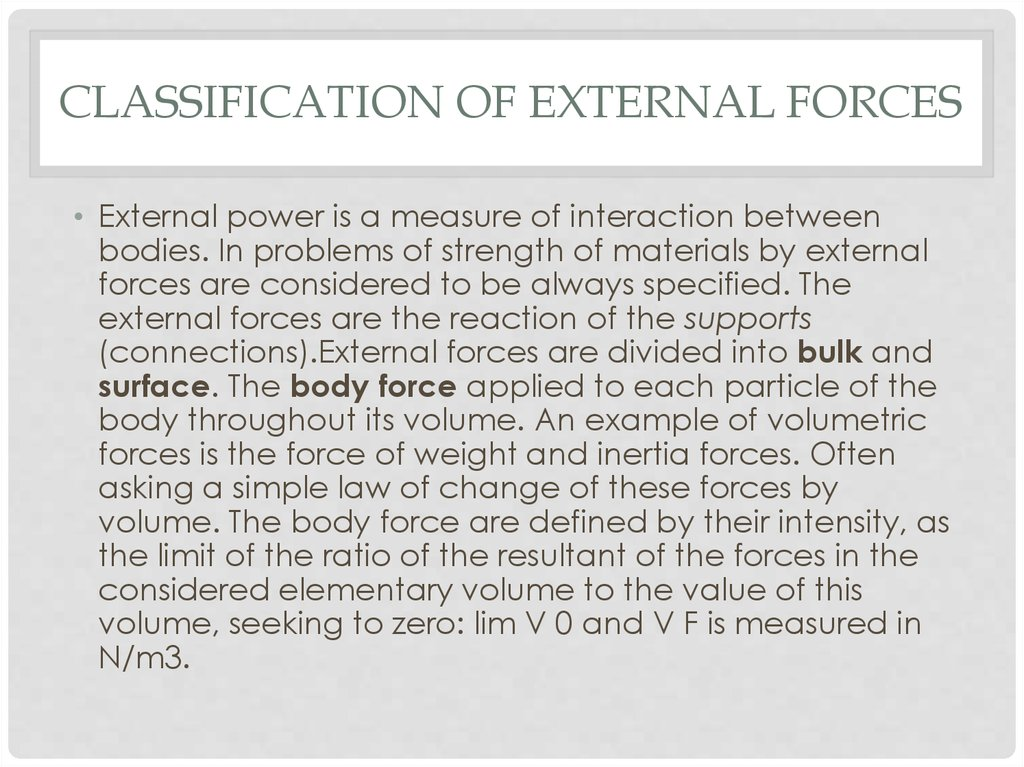Similar presentations:

# Classification of external forces. Method of section. Stress

## 1. Classification of external forces.method of section.Stress

CLASSIFICATION OF EXTERNAL
FORCES.METHOD OF
SECTION.STRESS

## 2. introduction

INTRODUCTION
• Resistance of materials - the section of technical
mechanics that studies experimental and
theoretical basis and methods of calculating the
common elements of different constructions, under
the influence of external loads on strength, rigidity
and stability, taking into account requirements of
reliability, efficiency, manufacturability, ease of
transportation and installation as well as safety
during operation.

## 3. Classification of external forces

CLASSIFICATION OF EXTERNAL FORCES
• External power is a measure of interaction between
bodies. In problems of strength of materials by external
forces are considered to be always specified. The
external forces are the reaction of the supports
(connections).External forces are divided into bulk and
surface. The body force applied to each particle of the
body throughout its volume. An example of volumetric
forces is the force of weight and inertia forces. Often
asking a simple law of change of these forces by
volume. The body force are defined by their intensity, as
the limit of the ratio of the resultant of the forces in the
considered elementary volume to the value of this
volume, seeking to zero: lim V 0 and V F is measured in
N/m3.

## 4.

• The surface forces are divided into concentrated and
distributed.Considered concentrated force applied to a
small surface, whose dimensions are small compared to
body size. However, when calculating stresses near the
zone of application of the force load should be
considered distributed. To concentrated loads include
not only force but also a pair of forces, an example of
which can be considered load of a wrench when
tightening the nuts. Concentrated efforts are measured
in kN.Distributed loads are distributed along the length
and square . To distributed loads include the pressure of
liquid, gas, or other body. Distributed forces are
measured, as a rule, in kN/m (distributed over length)
and kN/m2 (distributed by area).

## 5.

• All external loads can be divided into static and
dynamic.Static loads are considered in the application
process which the resultant of the inertia forces are small
and can be neglected. If inertia forces are high (for
example earthquake) loads are dynamic. Examples of such
re-variables. Suddenly applied loads transferred to the
structure fromfull its value (for example the pressure of the
wheels of the locomotive, a part of the bridge).Shock loads
occur when a rapid speed change of the osculating
elements of a design, e.g." when you hit women falling
weight of a pile during driving. Re-variable loads act on the
structure of repeating a significant number of times. Such,
for example, re-pressure steam, alternately tensile and
compressive piston rod and the connecting rod of a steam
engine. In many cases, the load is a combination of several
types of dynamic effects

## 6.

Method of sections
F3
z
B
Mz
П
F1
F3
Qz
F4
F4
N
A
П
F2
y
Qy
Mx
My
x
6

## 7.

• A method of sections involves the mental dissection
of the body of the plane and consider the
equilibrium of any one of the clipping partsA
method of sections allows to determine the
magnitude of the internal force factors in the
section, but does not allow to establish the
distribution of internal forces over the cross section.
To assess the safety, you must determine the
amount of force falling on any point of the cross
section.

## 8.

Method of sections
F1
A – elementary area
F2
F
f cp
– medium voltage
A
F – full voltage on ale.
lim
f site
A
fср
A
F
F
f
L
2
H
;
2
м
Н
1 2 1Па;
м
8

## 9.

Method of sections
Lay full stress on the
components:
x
y
sx
tyx
tzx
z
sx
– normal stress
A
t zx ,t yx
– tangent voltage
9

## 10.

• Voltage is the intensity of the action of internal
forces in the body, that is, tension is an internal
force per unit area. By its nature, stress is the surface
load occurring on the internal surfaces of the
contact parts of the body. Voltage, as well as the
intensity of the external surface load, is expressed in
units of force per unit area:PA=N/m2 (MPa = 106
N/m2, kgf/cm2=98 066 PA ≈ 105pa, TF/m2, etc.).

## 11. Will rossicum body of arbitrary cross-section

WILL ROSSICUM BODY OF ARBITRARY
CROSS-SECTION
Select a small area ∆A. the Internal stress acting
on it are denoted by ∆R ->. Full secondary
voltage of the pad R =∆R ->/∆A . Find the limit of
this ratio when ∆A ->0 . This will be a full voltage to
(the point) of the body.

## 12.

p =lim A
0 A R
The total voltage p->as the resultant of the internal forces applied on
the elementary Playground is a vector quantity and can be
decomposed into two components: perpendicular to the ground –
normal stress σn and tangential to the pad – shear stress Tn. Here n is
the normal to the designated site 1. Shear stress, in turn, can be
decomposed into two components parallel to the coordinate axes x,
y, associated with the cross-section is nx ny. In the name of shear stress,
the first subscript indicates the normal to the area,the second index is
the direction of shear stress.

## 13. Stress tensor

STRESS TENSOR
In the analysis of stresses in the vicinity
of the considered point stands infinitely
small volume element (a box with sides
dx, dy, dz), at each side of which are,
in the General case, three voltage, for
example, for faces perpendicular to xaxis (site x) – σx xy xz Stress components
on three perpendicular faces of the
elements form a system of stresses is
described by the special matrix –stress
tensor

## 14.

• Here the first column represents stress components at the
sites,normal to the axis x, the second and the third to the
y-axis and z respectively.When you rotate the
coordinate axes coinciding with the normals to the faces
selectedelement stress components change. Rotate the
selected item around the coordinate axes, we can find
the position of the element in which all shear stresses on
the faces of the element is equal to zero.The ground on
which shear stresses are zero, is called the main
platform.Normal stress on the main floor is called the
main voltageThe normal to the main site is called the
principal axis of the stress .Each point can hold three
mutually perpendicular main site.When rotating the axes
changes the components of the stress, but does not
change the stress-strain state of the body (VAT).

## 15.

Stress and strain
F
F
The Bernoulli hypothesis (the hypothesis of flat sections):
Cross-section deformation is not curved, i.e. remain flat ->
tensile-compression all the longitudinal fibers extend to the
ode and the same size.
15

## 16.

Напряжения и деформации
"to" strain
b
b1
«after deformation
l
l - the rod extension,
l
b = b1 – b – the narrowing of the rod
Are relative deformation:
l
l
- longitudinal
deformation
b
b
*
- transverse
deformation
16

## 17.

Stress and strain
In 1676 Robert Hooke experimentally determined
sx = E
E – modulus of elasticity
([E] = МПа)
Steel: E ~ 2·105 МПа
Copper: E ~ 1·105 МПа
Concrete: E ~ 104 …105 МПа
Aluminum: E ~ 7·104 МПа
17

## 18.

Stress and strain
In the cross section of the rod:
N s x A
A
А
sx
N
N s x dA т.к.s x const s x A
A
N normal stress in the cross
section of the rod
sx
A
Nl
l
EA
Hooke's law for elongation
stiffness of a rod under tension
18

## 19.

Stress and strain
Condition tensile strength:
s max
N max
R
A
или
[s ]
R – the calculated resistance
[s] – allowable stress
19